# Artificial Intelligence – 1. Perceptrons

## The simplest form of artificial intelligence

Perceptrons are a probabilistic model for information storage and organization within the brain.  They can be trained to classify linearly separable patterns.  Perceptrons can be organized into a MISO (Multiple-Input, Single-Output) “feed-forward” network that takes in binary inputs and gives binary outputs.  A single perceptron is shown below.Simple Perceptron Model

In the model,are individual inputs (from outputs of other neurons or from direct input),are weights,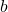is the bias,is the output from the perceptron before the activation function, and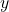is the final output from the perceptron.

The output of the perceptron is calculated by adding each of the inputs times the input’s weight, along with the bias..  This value,, is then passed through the activation function to find the final output.The activation function for a simple perceptron network is usually the hardlim() function, which outputs 1 if the input is positive and 0 if the input is negative.Hardlim() function

Alternatively, the hardlims() function can also be used as the activation function, which outputs -1 if the input is negative and 1 if the input is positive.

The output of the perceptron can be calculated in matrix format:Note that the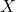matrix needs the 1 in the first position to multiply the bias.  This could be -1 if desired, but unnecessary because the biascan be positive or negative.

## Logic gates with perceptrons

A perceptron can act as logic gates.  For example, a 2-input perceptron can act as an “and” operator with the following weights:.1-Neuron perceptron neural network acting as AND gate.

This network is even fault tolerant – input values do not need to be exactly 0 or 1.Fault tolerance of AND perceptron

The output of the perceptron can be graphically displayed as below.  The horizontal axis corresponds to input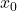and the vertical axis corresponds to input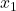.  The outputs are plotted in their propercoordinate and displayed as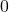or.  Any combination of inputs will fall in the shaded areas needs an output of 1, while any other combinations need an output of 0.  Because there exists a line between on which the output classes can be separated, the inputs and outputs are linearly separable.  Note that the equation for the decision boundary is.Plot of perceptron inputs and outputs.

## Multi-layer networks

A single-layer perceptron can only classify into 2 classes that are linearly separable.  A multi-layer network is needed for patterns that are not linearly separable.  These multi-layer networks contain a hidden layer.Multi-layer feed forward perceptron neural network.

These multi-layer networks can be used to classify inputs more complexly.  Take the XOR function – it is linearly inseparable and needs a hidden layer.The network is able to classify the linearly inseparable inputs by mapping the “input space” to a “feature space” of the hidden layer.  The feature space from the output neuron (Neuron 5) for the XOR gate is shown below.